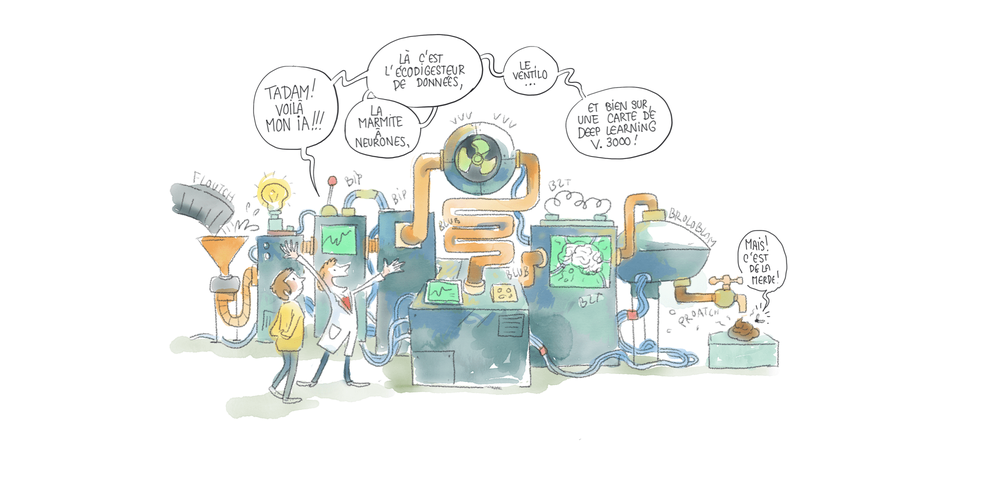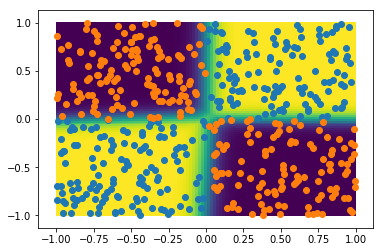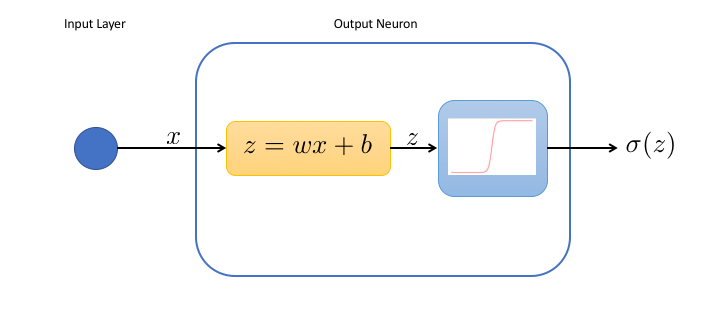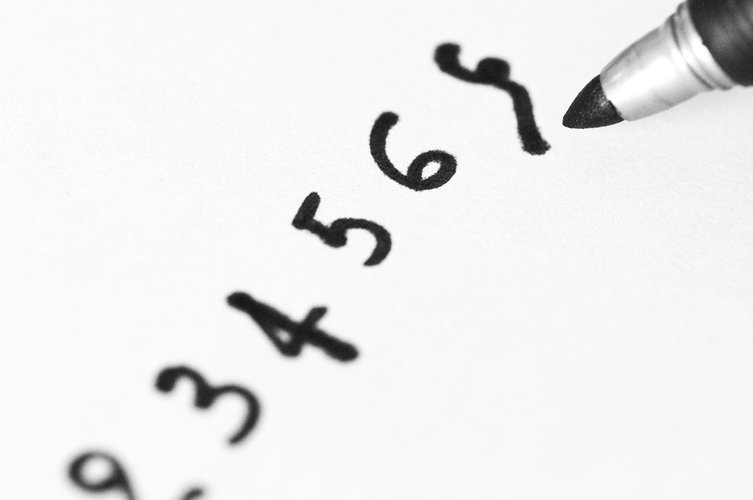# The Data Frog neural-network## Overfitting Illustrated

Overfitting is one of the most important issues in machine learning. Here, it's illustrated in a small 2D classification problem.## Logistic Regression vs Neural Network: Non Linearities

Back to the logistic regression, aka the 1-neuron network! Here we see that it works well in 2D as well for linear problems. We also find out that it can be necessary to introduce non linearity by adding hidden layers## The 1-Neuron Network: Logistic Regression

So far we've used neural networks as a black box. Today, we're opening the box. To keep it easy, we'll do it for a very, very simple neural network, with a single neuron.## First Neural Network with Keras

Keras is the easiest and most powerful way to deal with neural networks in python. Give it a try by training your first neural net to classify handwritten digits## Handwritten Digit Recognition with scikit-learn

In this tutorial, we apply supervised machine learning to train a neural network to classify images of handwritten digits into the following categories: 0, 1, 2, ... , 9. If you're just getting started with machine learning, this image recognition tutorial is for you!

• 1